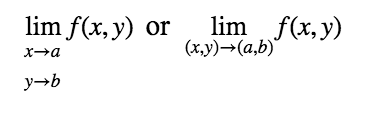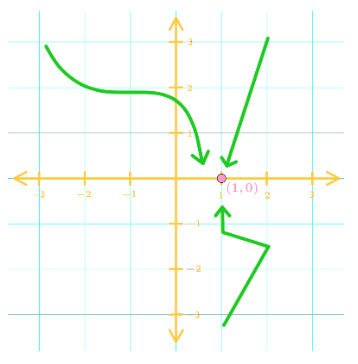# Limits and continuity of multivariable functions

### Limits and continuity of multivariable functions

#### Lessons

Notes:

Notation for a Limit of 2 Variable Functions Suppose we want to take the limit of $x \to a$ and $y \to b$ of f(x,y). Then we write this notation as:In a sense, we are trying to approach to the point $(a,b)$ in the function $f(x,y)$ without being at that point.

Limit of Continuous 2 Variable Functions
A function $f(x,y)$ is continuous at $(a,b)$, then:

$\lim\limits_{(x,y) \to (a,b)} f(x,y) = f(a,b)$

In other words, if the function is continuous at $(a,b)$, then plug in $x=a$ and $y=b$ to evaluate the limit!

Ways to see if it is continuous at $(a,b)$:
1. Plug $(a,b)$ into the function and see if the value is undefined. If it is not undefined, then it is continuous.
2. If the function has a denominator, plug $(a,b)$ into the denominator and see if it gives 0. If it is not 0, then the function is continuous.

Visualizing the Limit
There are many paths to approaching a specific point. For example, look at this graph:There are an infinite number of paths into approaching the point $(1, 0)$.
One thing to note: If all paths lead to the same value, then the limit is equal to that value.

What if the Function is Discontinuous at (a,b)?
You want to take different paths to $(a,b)$ and see if the limits are different. For example, one path can be $y=x^2$ and the other path can be $y=x$. If evaluating these paths with limits give different values, then the limit does not exist! We will show an example of this in the video.
• Introduction
Limits & Continuity of Multivariable Functions Overview:
a)
Notation for a Limit of 2 Variables Functions
• The limit of $f(x,y)$ as $x \to a$, $y \to b$
• What Does This Mean?
• Approaching the point $(a,b)$

b)
Visualizing the Limit
• Can take infinite paths
• Do all paths give the same value?
• If it does, then the limit is that value.

c)
Limit of Continuous 2 Variable Functions
• If continuous, then plug $(a,b)$ into the function
• How do we know it's continuous?
• 2 Ways to See Continuity

d)
What if the Function is Discontinuous?
• Step 1: See if function is discontinuous
• Step 2: Find a path
• Step 3: Find a second path
• Compare paths

• 1.
Finding the Limit
Evaluate the following limit:

$\lim\limits_{ (x,y) \to (2,2) } \frac{x-y}{y-x}$

• 2.
Evaluate the following limit:

$\lim\limits_{(x,y,z) \to (e,3,4)} \frac{ln(x^2) + \sqrt{y^2 + z^2}}{e^x - 2y + z}$

• 3.
Evaluate the following limit:

$\lim\limits_{(x,y) \to (4,1)} \frac{xy^2 - 4y^3}{x^2 - 16y^2}$

• 4.
Finding Discontinuous Limits
Evaluate the following limit

$\lim\limits_{(x,y) \to (0,0)} \frac{x+y}{x-y}$

• 5.
Evaluate the following limit

$\lim\limits_{(x,y) \to (0,0)} \frac{x^3 - y^6}{xy^2}$